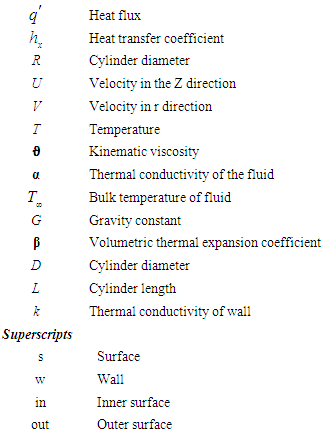﻿ Numerical Investigation on Effect of Wind and Gas Injection on Indirect Dry Cooling Towers: A Case Study of Shaheed Rajaee Power PlantAmerican Journal of Fluid Dynamics

p-ISSN: 2168-4707    e-ISSN: 2168-4715

2018;  8(3): 96-104

doi:10.5923/j.ajfd.20180803.03### Numerical Investigation on Effect of Wind and Gas Injection on Indirect Dry Cooling Towers: A Case Study of Shaheed Rajaee Power Plant

Reza Amini1, Mehdi Kashfi1, Mohsen Amini2

1Department of Mechanical Engineering, University of Tabriz, Tabriz, Iran

2Department of Mechanical Engineering, K. N. Toosi University of Technology, Tehran, Iran

Correspondence to: Mohsen Amini, Department of Mechanical Engineering, K. N. Toosi University of Technology, Tehran, Iran.
 Email: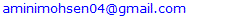Abstract

Numerical study of effective parameters on natural convection heat transfer in a dry cooling tower is carried out by using the commercial software of Ansys-Fluent. The modelled dry cooling tower has the same geometry as in Shahid Rajaee power plant in Iran. For a particular performance of the cooling tower, numerical and experimental results are compared, and suitable algorithm and turbulence model are determined. Results show that k-e-RNG full buoyancy effect model is the most accurate approach for modeling the turbulence and COUPLED algorithm is the best for solving for the coupling between pressure and velocity fields. Grid independency is validated, and the best numerical grid is selected. Due to presence of external flow, domain independency is also checked, and the best domain is selected. Effect of wind and force convection on cooling process is also studied. At the end, effect of high velocity air injection on increasing momentum and efficiency of the cooling process is studied. At moderate atmospheric condition, air injection slightly improves the amount of heat transfer, but it can reinforce the flow in unstable conditions like stormy weather and thus sustain the cooling process.

Keywords: Computational fluid dynamics (CFD), Natural convection, Heat transfer, Dry cooling tower

Cite this paper: Reza Amini, Mehdi Kashfi, Mohsen Amini, Numerical Investigation on Effect of Wind and Gas Injection on Indirect Dry Cooling Towers: A Case Study of Shaheed Rajaee Power Plant, American Journal of Fluid Dynamics, Vol. 8 No. 3, 2018, pp. 96-104. doi: 10.5923/j.ajfd.20180803.03.

### 1. Introduction

Natural convection is a heat transfer mechanism that happens due to gravity and differences in air density. Density difference could be caused by existing temperature gradient and fluid composition. Natural convection depends on surface geometry, temperature difference, and thermo-physical properties of the fluid. Convective heat transfer can be categorized depending on the nature of flow. In force convection, flow is created by external sources like fans, pumps or atmospheric winds [1-6], which makes it controllable and more efficient for applications where temperature should be monitored such as engine cooling systems . In contrast, natural or free convection occurs due to buoyancy force, which is caused by density difference in air parcels. Free convection in cylindrical chamber has been an interesting subject for scientists and engineers due to numerous applications in various fields. Cylindrical objects have numerous applications in industry including exhaust towers, cooling towers, tornado simulators [8, 9], tubes, thermal rods, coils, bioreactors, wires, etc. For a tall vertical cylinder, natural convection and fluid flow are studied analytically and numerically by Eckert  and Ozisik  and Ramezani et al. . Natural convection in horizontal cylinders can be simplified by considering it as a 2D problem. This approach can be applied to vertical cylinders. Asymmetrical solutions of this problem are well studied analytically [13-15] and numerically [16, 17] in the literature. In case of a thin vertical cylinder, a fuel cell in a research reactor for instance, there is only one numerical solution provided by Lee et al. , which needs more scrutiny and validation. Badruddin et al.  studied natural convection in a porous ring-shaped object. The optimized use of turbulence for natural convection on a horizontal cylinder is studied by Bassam and Abu-Hijleh . Over the past decades, accurate numerical techniques have been used for different engineering and academic applications such as cooling system of turbomachinery [21-23], heat transfer [24, 25], wind energy [26-28], dynamic systems [29, 30], ocean wave energy convertor such as new type “Searaser” [31-32], and power generation systems [33-36] as well as accurate experimental techniques using image processing [37-39]. Numerical study of natural convection around a set of 2 isothermal horizontal cylinders is studied by Chouikh et al. , they scrutinized the effect of geometry, velocity and temperature of the fluid on the heat transfer, and they showed that the heat transfer of the top cylinder increases with the Rayleigh number for the same spacing. Yousefi and Ashjaee  studied natural convection from a set of isothermal oval vertical cylinders experimentally. This study showed the impact of Rayleigh number, cylinders separation distance, and cylinder position in the array on the free convection heat transfer for any individual cylinder in the array. Natural convection has been subject of active study in various fields. Davani et al. [44, 45] developed a solar energy driven distillation still that uses natural convection as for water treatment. Convection on cylindrical objects have also been used studied in gas contamination removal techniques .
In the current study, heat transfer over a dry cooling tower has been simulated using a CFD model. By comparing the results from the simulations and experimental data, the best models for simulation are chosen. After validating simulated results, impact of wind velocity and high velocity air injection on heat transfer are investigated. Finally a comparison between results of different conditions is presented to investigate usefulness of high velocity air injection on the cooling rate.

### 2. Description

#### 2.1. Importance of Cooling Towers

In a dry cooling tower air is used as the coolant. In this type of cooling process, hot and cold fluids are not directly in contact. Instead, hot fluid which can be liquid, or vapor passes through a set of finned tubes and heat transfer process occurs as air flows over the tubes. Air flow can be natural due to free convection or it can be forced by using a suction or blower fan. Since there is no need for makeup water in dry cooling towers, they are considered cost-effective and favorable alternative in areas with limited water resources. The cooling mechanism in dry cooling towers is the same as heat exchangers where heat is transferred due to convection unlike evaporative cooling systems. Therefore, the minimum achievable temperature is ambient dry-bulb temperature (DBT) and not the wet-bulb temperature (WBT). DBT is usually higher than WBT; thus, the efficiency of a dry tower is higher in dry rather than humid days.
Direct dry cooling tower is one of the most common applications of dry cooling processes that acts like car radiator. In this method, water vapor that leaves turbine, enters a series of finned tubes where it cools down with the flow of cooler air on the outer side of the tubes. In direct dry cooling towers, which are called air condenser, vapor tubes are in an A shape figure. Water vapor is condensed and directed to steam production cycle. Dry cooling towers do not have undesirable effects like fog production which occurs in wet cooling towers.

#### 2.2. Disadvantages of Dry Cooling Tower

As previously mentioned, cooling process in dry towers is a function of ambient DBT which means that the minimum achievable fluid temperature is ideally equal to the ambient temperature. Therefore, since DBT is often more than WBT, dry cooling towers perform weaker than wet cooling towers in warm weather. Moreover, since there is a partial vacuum in dry cooling towers, air leakage is a major problem.
Since the cooling process is based on convection in dry cooling towers, a big cross-sectional area is needed; therefore, the tower is much larger compared to wet cooling towers and as a result higher capital investment is needed.

#### 2.3. State of the Problem

In this paper effect of various factors on heat transfer process in a dry cooling tower will be studied.
Geometry of the problem: A dry cooling tower in Shahid Rajaee power plant that is located 25 km away from Qazvin, Iran is selected for the simulation. The dimensions and other characteristics of the hyperbolic tower are as follows: Tower height is 125 m lower diameter is 110 m, upper diameter is 62 m, number of triangular radiators is 284, the height of the radiators is 20 meters from the ground, width of the radiators is 1.5 m each, and radiators have placed in an arrow configuration with outer angle of 60°. The injector has a diameter of 8 m which is 12 m tall, and it is located at height of 52 m. The domain is 1375 m tall and has the diameters of 1430 m. The model geometry and computational domain can be seen in Fig. 1 and 2.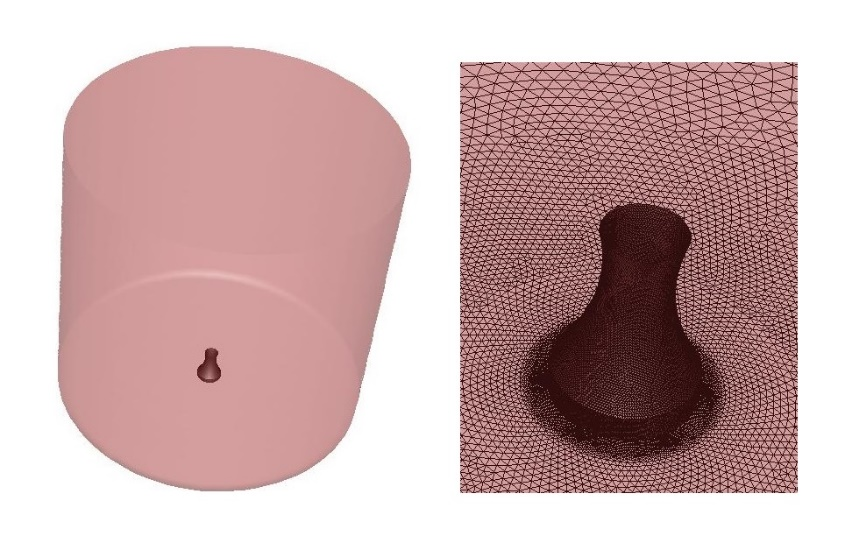Figure 1. Geometry of studied dry cooling tower and simulation domain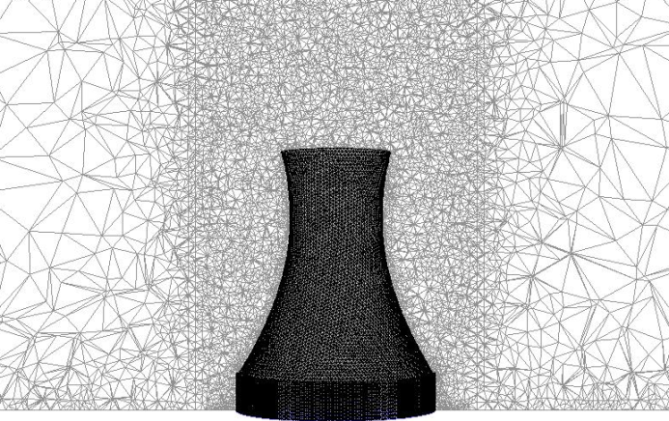Figure 2. Mesh for the simulation

### 3. Solution Method

#### 3.1. Mesh Generation

Domain Independency: In study of external flow around Heller dry cooling tower domain independency analysis is crucial. It this analysis, domains of different sizes should be examined to find the smallest domain where the increase in its size does not have any influence on the solution. The domain needed in this problem is a cylinder with height of H (m) and diameter of D (m). Both dimensions will be increased, while D/H is fixed. The increasing factors would be chosen as a function of tower’s height and the biggest diameter. Results of domain independency can be seen in Table, where Di is the domain’s diameter and D0 is tower’s biggest diameter.
 Table 1. Domain independency evaluation for the simulation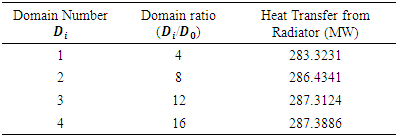Grid Independency: As it can be seen in Fig. 2, tetrahedral mesh grid is used for discretizing the domain for the simulation. Before discussing the results from simulation, grid independency should be evaluated. In grid independency analysis, further decrease in the mesh grid sizing of the independent grid does not change the solution with a specific accuracy. In Table 2, 4 different mesh grid sizes are considered. First and second mesh grids changed the results drastically, but the third one can be considered as the principal mesh grid for this study since the further decrease in mesh sizing (increase in the number of computational cells) does not change the results significantly (less than 0.01% change in radiator heat transfer).
 Table 2. Number of cells and corresponding simulation result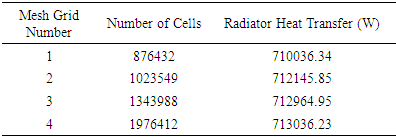#### 3.2. Governing Equations

Boussinesq approximation will be used in which all the properties of the fluid except the density in the buoyancy term in momentum equation are fixed. This assumption is valid when the temperature difference between wall and the fluid is less than 20 degrees Celsius. Otherwise, the ideal gas law assumption will be used. Based on these assumptions, governing equations for steady-state heat transfer are mass, momentum, and energy conservation. Governing equations in cylindrical coordinate can be written as follows: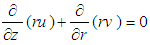(1)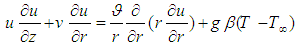(2)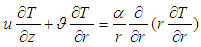(3)
where r is radial coordinate,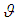is kinetic viscosity, u and v are velocity component in r and θ directions, respectively. In natural convection problems, the driving force is caused by density differences which is a function of temperature gradient. Hence, identifying temperature field is essential for solving the velocity field. Consequently, momentum and energy equations should be solved simultaneously. The main flow in this type of heat exchangers is natural convection heat transfer or in other words, buoyancy-driven flow. Principal reason behind natural convection heat transfer process is reduction in density due to increase in temperature; Thus, warmer fluid will be replaced by colder and consequently lighter fluid will move against gravity. For this reason, a function for density variation and change in temperature should be used. Ideal gas law and Boussinesq equation are used for this purpose. Normally when the temperature difference is less than 20 degrees, Boussinesq equation is used to model density variations with temperature change. Using the ideal gas law decreases total run time in simulation, but occasionally has higher error. In this study, both methods are used for comparison to experimental data and results are presented in Table 3.
 Table 3. Heat Transfer as a function of different models for simulating the density in a buoyant flow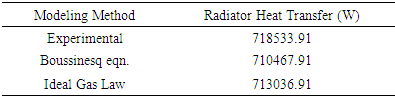Boussinesq equation is in the form of: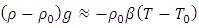(4)
where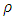and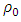are density and reference density, g is gravitational acceleration,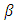is thermal expansion coefficient,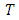and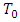are temperature and reference temperature. Coefficient of thermal expansion in Boussinesq equation is 0.0003331 (1/T) at the room temperature. Ideal gas law is in the form of: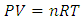(5)
where P is pressure, V is volume, n is amount of substance of the gas, T is temperature, R is gas constant.

### 4. Numerical Model Validation and Details

Before preforming the CFD simulation in any study, the accuracy of the appropriate algorithm for simulation should be verified. This will be done using comparison between experimental and numerical data. In the current simulation, geometrical data and performance of the dry cooling tower of Shahid Rajaee power plant is used for validation. In this study, coupling algorithms for pressure and velocity variables are used. Despite the small difference in the values between two methods, coupled algorithm has lowest error as it can be seen in Table 4 and hence, all the computations will be carried out using this method.
 Table 4. Sensitivity analysis of various pressure-velocity coupling models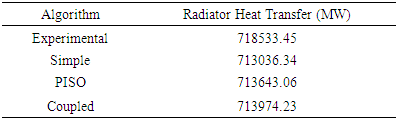Properties of Operating Fluid: Operating fluid is air with the density as a function of temperature;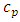is 1006.43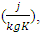thermal conductivity is 0.0242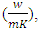viscosity is 1.79e-5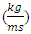and coefficient of thermal expansion is 0.00333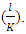Modeling Turbulence: 4 different turbulence models are used in this study. Results are presented in Table 5.
 Table 5. Sensitivity analysis of different turbulence models for a buoyant flow (heat flux of radiator)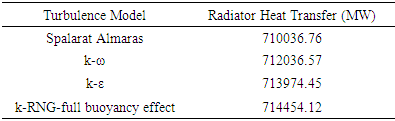Boundary Condition: Boundary conditions used for this problem include pressure outlet at the top of the tower with the ambient temperature of 298 K and no-slip wall condition; velocity inlet for the injector with the ambient temperature. Boundary condition for the domain boundaries is pressure outlet without any wind. In presence of wind it is divided to two sections with a velocity inlet and a pressure outlet. The walls of the radiator are isothermal. The fluid in radiator has initial temperature of 318 K. It should be also noted that the walls near base of the tower are also included to mimic use of wind breaks and thus wind cannot penetrate the tower from the bottom.
Solution Methods: PRESTO algorithm is used for calculation of pressure and velocity fields and second order upwind discretization scheme is used for solving momentum and energy equations. The Convergence Absolute Criteria (CAC) for momentum and continuity residuals is 0.001 and for energy equation is 1e-6. Further validation of the numerical scheme used in current study is presented in Gharraei et al. . Other multiphysics software such as Abaqus which offers a variety of user friendly subroutines may also be used for such simulations [48, 50].

### 5. Results and Discussions

Results for different cases are presented. The first case is performance of tower without any wind or air injection. The amount of heat transfer is presented in Table 6. The streamlines of the air in these three cases are shown in Fig. 3.
 Table 6. Effect of wind speed on heat transfer from radiators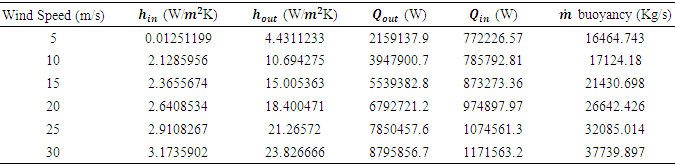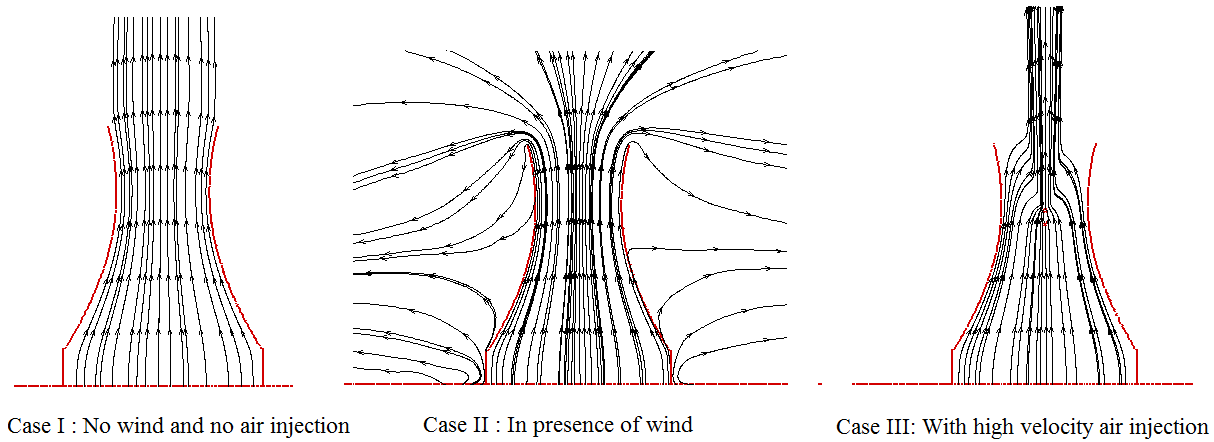Figure 3. Streamlines of the air inside the tower in different cases
Effects of wind speed on the rate of cooling: The flow in natural convection heat transfer processes or buoyancy-driven flows is in opposite direction of gravitational force. When the flow moves in the same direction of natural convection (opposite of gravity) due to injection, maximum heat transfer occurs. However, since such cooling towers are in open space and most of the times outside the city area, the efficiency changes due to environmental factors such as wind and rain. Pathlines of the hot air flow are shown in Fig. 4 without and in presence of wind. Effect of 5 different wind speeds on the performance of dry towers is studied in this paper. The comparison between air velocity inside the tower with and without the presence of wind in the domain are shown in Fig. 5. Contours of velocity in tower for various wind speeds are shown in Fig. 6 and the changes in temperature, static pressure, density, and velocity inside the tower can be seen in Fig.7. The parabolic behavior of plots could be explained by the shape of the tower. Since the geometry of the tower is such as a converging-diverging nozzle and given the fact that the fluid is considered incompressible, pressure has a decreasing-increasing behavior. Effect of wind speed on heat transfer from radiators is presented in Table 6.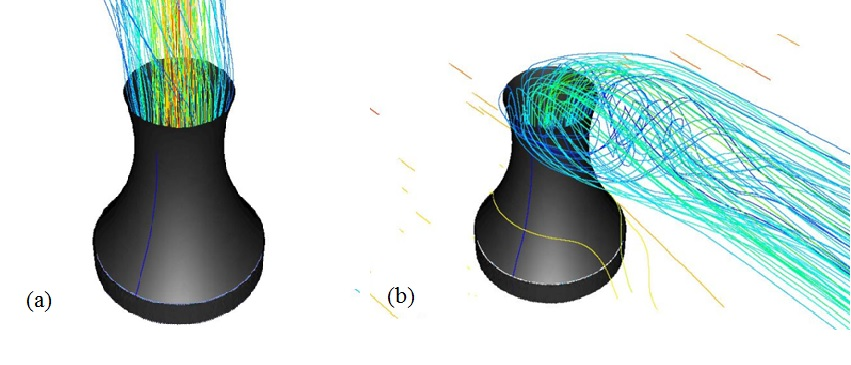Figure 4. Pathlines of hot air from radiator (a) with no wind and (b) in presence of wind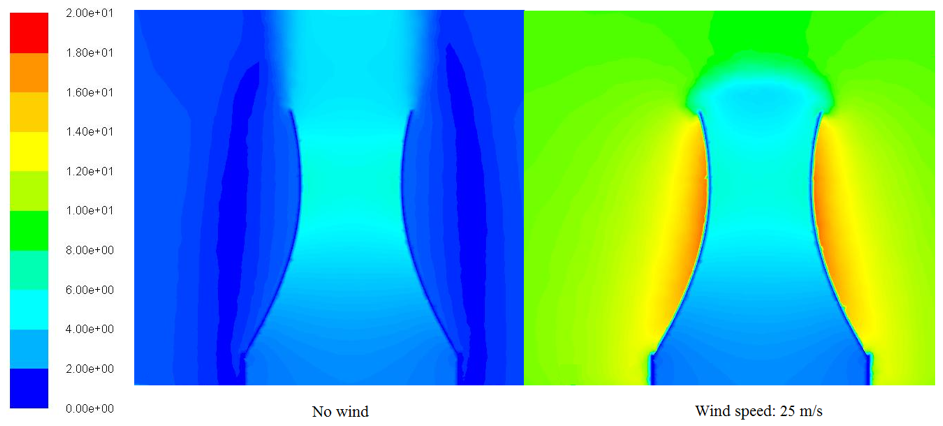Figure 5. Contours of velocity magnitude (m/s) in two different cases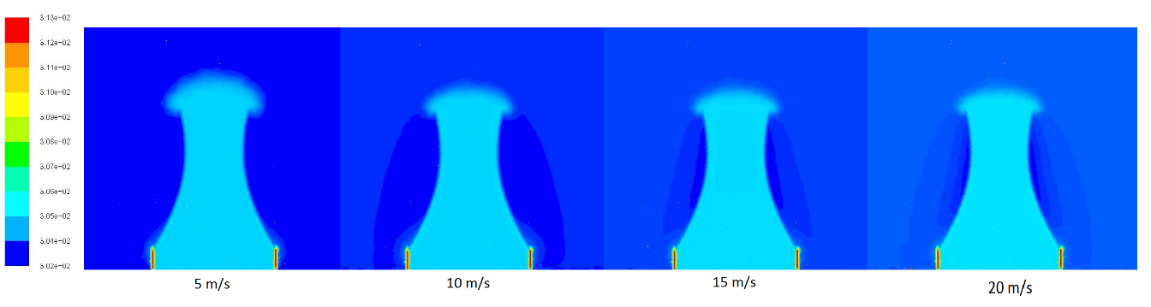Figure 6. Contours of velocity magnitude (m/s) for 4 different wind speeds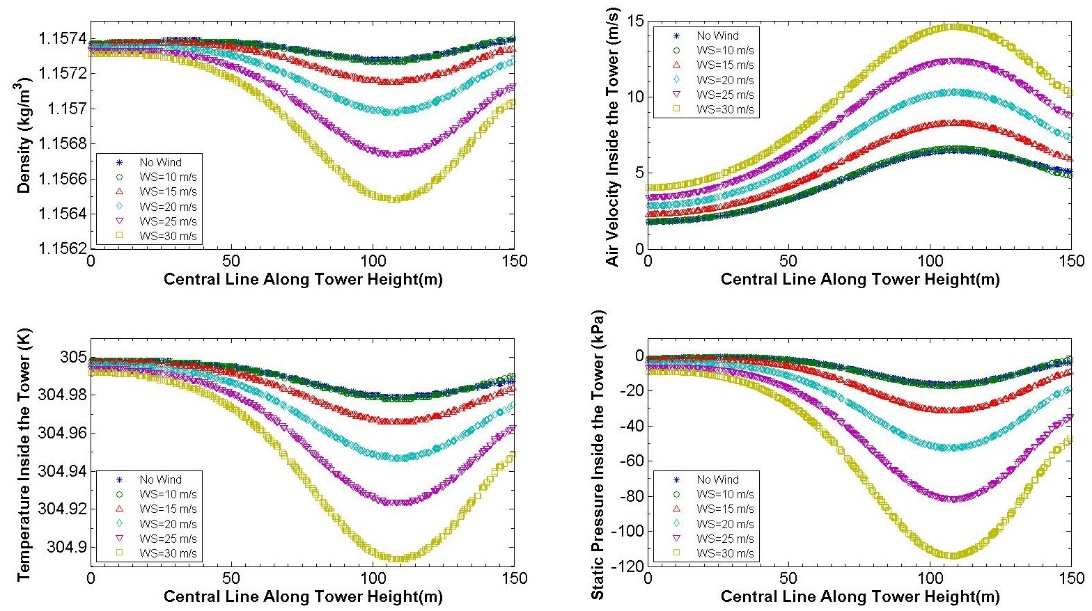Figure 7. Effect of various wind speeds on performance of tower
Effect of high speed air injection on efficiency improvement: The main reason for movement of the fluid in natural heat transfer is buoyant flow of the warm fluid. At low temperatures, density variation and consequently the velocity of buoyant flow is low. Since the buoyant flow has low momentum, any external disturbance such as vortex shedding due to turbulent flows changes the flow direction and as a result, the efficiency decreases. For reinforcing the buoyancy, increasing the momentum, and thus increasing the efficiency, air can be injected outside of the tower at high speeds.
Contour and vector of velocity inside the tower for air injection at 20 m/s is shown in Fig. 8 and Fig. 9 respectively and effect of air injection on heat transfer from radiators is presented in Table 7. Note that in both Table 6 and 7,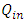is the amount of heat being transferred from inner surface of the radiators to the air inside the tower. It should be noted that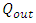is the amount of heat transfer from radiator’s outer surface to the air outside the tower.
 Table 7. Effect of high velocity air injection on heat transfer from radiators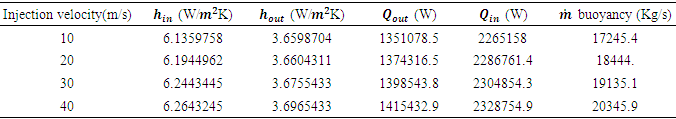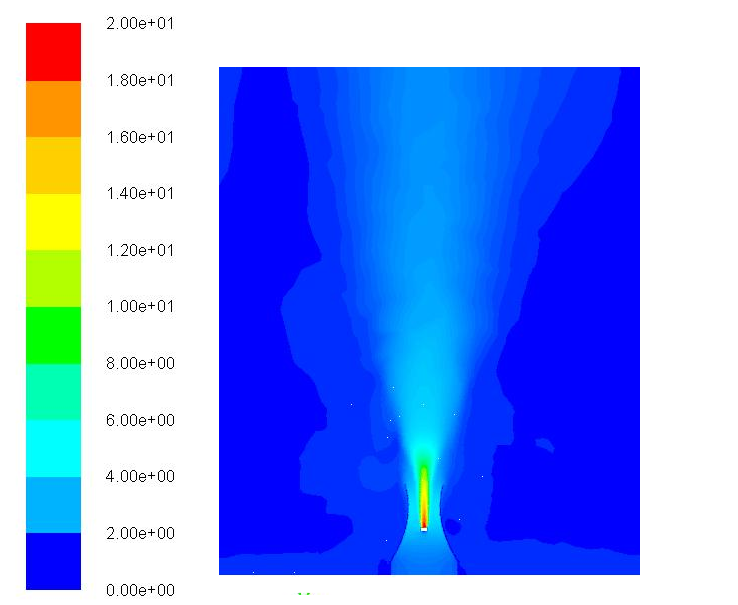Figure 8. Contour of velocity magnitude (m/s) with high velocity air injection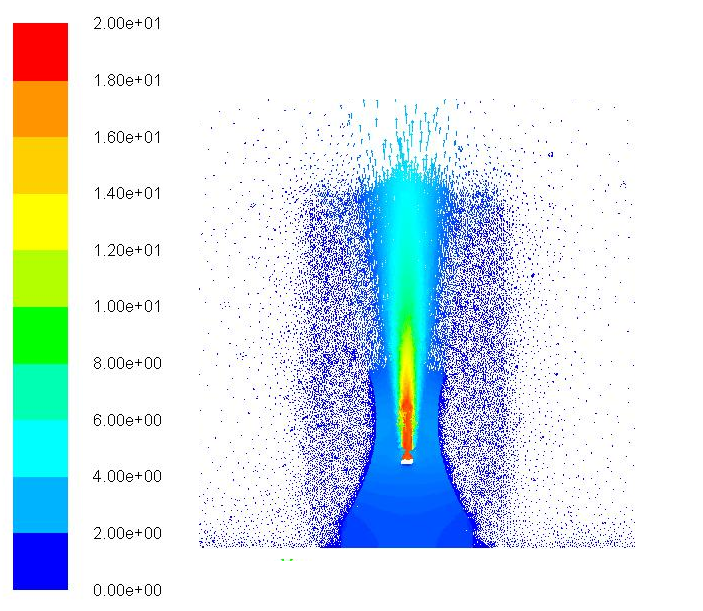Figure 9. Velocity vectors for high velocity air injection

### 6. Conclusions

In this study, 3-D CFD simulation of a dry cooling tower is performed to investigate effects of different parameters on natural convection heat transfer in a dry cooling tower. Different algorithms and turbulence models are used and the best ones are selected. COUPLED algorithm for coupling pressure and velocity field shows the best results for the simulations. Modeling buoyancy for radiator’s heat transfer rate using ideal gas law shows more consistency with experimental data rather than Boussinesq equation. In addition, among different RANS turbulence models used, k-ε RNG-full buoyancy effect is the best for simulating buoyancy. Finally, effects of wind speed and high velocity air injection are investigated. As it could be seen in Table 6, as the wind speed increases the pressure gradient in the tower increases and consequently air velocity increases. Increase in velocity inside the tower results in higher mass flow rate and higher convective heat transfer. After studying effects of high velocity air injection, it turns out that the performance of cooling tower is slightly improved compared to no wind conditions as in can be seen in Table 7. The improvement however, is not as much the case with wind and no injection. This means that air injection could be used to reinforce the flow in conditions with high turbulent flows around tower i.e. unstable weather like rain or storm in which wind direction changes a lot. Doing experimental investigation of all different cases is difficult since building these towers are expensive and studying this type of huge structures are not easy. Hence, CFD methods can be used to study cooling dry towers and to find probable solutions for increasing their efficiency.

### Nomenclature